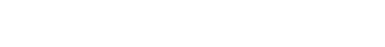## 缘起

DeepFM 不算什幺新技术了，用 TensorFlow 实现 DeepFM 也有开源实现， 那我为什幺要炒这个冷饭，重复造轮子？

## 数据预处理## input_fn

```def_decode_tsv(line):
columns = tf.decode_csv(line,record_defaults=DEFAULT_VALUES,field_delim='\t')
y = columns
feat_columns = dict(zip((tfor tinCOLUMNS_MAX_TOKENS), columns[1:]))
X = {}
for colname, max_tokensinCOLUMNS_MAX_TOKENS:
# 调用 string_split 时，第一个参数必须是一个list，所以要把 columns[colname]放在[]中
# 这时每个 kv 还是'k:v'这样的字符串
kvpairs = tf.string_split([feat_columns[colname]], ',').values[:max_tokens]
# k,v 已经拆开, kvpairs 是一个 SparseTensor，因为每个 kvpair 格式相同，都是"k:v"
# 既不会出现"k"，也不会出现"k:v1:v2:v3:..."
# 所以，这时的 kvpairs 实际上是一个满阵
kvpairs = tf.string_split(kvpairs, ':')
# kvpairs 是一个[n_valid_pairs,2]矩阵
kvpairs = tf.reshape(kvpairs.values, kvpairs.dense_shape)
feat_ids, feat_vals = tf.split(kvpairs, num_or_size_splits=2, axis=1)
feat_ids = tf.string_to_number(feat_ids,out_type=tf.int32)
feat_vals = tf.string_to_number(feat_vals,out_type=tf.float32)
# 不能调用 squeeze, squeeze 的限制太多, 当原始矩阵有1行或0行时，squeeze 都会报错
X[colname+ "_ids"]= tf.reshape(feat_ids, shape=[-1])
X[colname+ "_values"]= tf.reshape(feat_vals, shape=[-1])
return X, y```

```def input_fn(data_file, n_repeat, batch_size, batches_per_shuffle):
for c, max_tokensinCOLUMNS_MAX_TOKENS:
pad_values[c +"_ids"] = -1# 0 is still valid token-id, -1 for padding
# no need to pad labels
dataset= dataset.map(_decode_tsv,num_parallel_calls=4)
ifbatches_per_shuffle >0:
dataset= dataset.shuffle(batches_per_shuffle * batch_size)
dataset= dataset.repeat(n_repeat)
iterator= dataset.make_one_shot_iterator()
dense_Xs,ys= iterator.get_next()
# ----------- convert dense to sparse
sparse_Xs= {}
for c, _inCOLUMNS_MAX_TOKENS:
for suffixin["ids","values"]:
k="{}_{}".format(c, suffix)
sparse_Xs[k] = tf_utils.to_sparse_input_and_drop_ignore_values(dense_Xs[k])
# ----------- return
return sparse_Xs, ys```

## 建立共享权重

field 就是 active_pkgs、install_pkgs、uninstall_pkgs 这些大类 ，是 DataFrame 中的每一列
feature 就是每个 field 下包含的具体内容， 一个 field 下允许存在多个 feature ，比如前面提到的 qq, weixin, taobao 这样的 app 名称。
vocabulary 对应例子中的“package 字典”。 不同 field 下的 feature 可以来自同一个 vocabulary ，即若干 field vocabulary

```classEmbeddingTable:
def__init__(self):
self._weights = {}
"""
:param vocab_name: 一个 field 拥有两个权重矩阵，一个用于线性连接，另一个用于非线性（二阶或更高阶交叉）连接
:param vocab_size: 字典总长度
:param embed_dim: 二阶权重矩阵 shape=[vocab_size, order2dim]，映射成的 embedding

:return: None
"""
linear_weight = tf.get_variable(name='{}_linear_weight'.format(vocab_name),
shape=[vocab_size,1],
initializer=tf.glorot_normal_initializer(),
dtype=tf.float32)
# 二阶（FM）与高阶（DNN）的特征交互，共享 embedding 矩阵
embed_weight = tf.get_variable(name='{}_embed_weight'.format(vocab_name),
shape=[vocab_size, embed_dim],
initializer=tf.glorot_normal_initializer(),
dtype=tf.float32)
self._weights[vocab_name] = (linear_weight, embed_weight)
defget_linear_weights(self, vocab_name):returnself._weights[vocab_name]
defget_embed_weights(self, vocab_name):returnself._weights[vocab_name]

defbuild_embedding_table(params):
embed_dim = params['embed_dim']# 必须有统一的 embedding 长度
embedding_table = EmbeddingTable()
forvocab_name, vocab_sizeinparams['vocab_sizes'].items():
returnembedding_table```

## 线性预测部分

```def output_logits_from_linear(features, embedding_table, params):
field2vocab_mapping= params['field_vocab_mapping']
combiner= params.get('multi_embed_combiner', 'sum')
fields_outputs= []
# 当前 field 下有一系列的 <tag:value> 对，每个 tag 对应一个 bias（待优化），
# 将所有 tag 对应的 bias，按照其 value 进行加权平均，得到这个 field 对应的 bias
for fieldname, vocabnameinfield2vocab_mapping.items():
sp_ids= features[fieldname +"_ids"]
sp_values= features[fieldname +"_values"]
linear_weights= embedding_table.get_linear_weights(vocab_name=vocabname)
# weights: [vocab_size,1]
# sp_ids: [batch_size, max_tags_per_example]
# sp_weights: [batch_size, max_tags_per_example]
# output: [batch_size, 1]
output= embedding_ops.safe_embedding_lookup_sparse(linear_weights, sp_ids, sp_values,
combiner=combiner,
name='{}_linear_output'.format(fieldname))
fields_outputs.append(output)
# 因为不同 field 可以共享同一个 vocab 的 linear weight，所以将各个 field 的 output 相加，会损失大量的信息
# 因此，所有 field 对应的 output 拼接起来，反正每个 field 的 output 都是 [batch_size,1]，拼接起来，并不占多少空间
# whole_linear_output: [batch_size, total_fields]
whole_linear_output= tf.concat(fields_outputs,axis=1)
tf.logging.info("linear output, shape={}".format(whole_linear_output.shape))
# 再映射到 final logits（二分类，也是 [batch_size,1]）
# 这时，就不要用任何 activation 了，特别是 ReLU
return tf.layers.dense(whole_linear_output,units=1,use_bias=True,activation=None)```

## 二阶交互预测部分```def output_logits_from_bi_interaction(features, embedding_table, params):
field2vocab_mapping= params['field_vocab_mapping']
# 论文上的公式就是要求 sum，而且我也试过 mean 和 sqrtn，都比用 mean 要差上很多
# 但是，这种情况，仅仅是针对 criteo 数据的，还是理论上就必须用 sum，而不能用 mean 和 sqrtn
# 我还不太确定，所以保留一个接口能指定其他 combiner 的方法
combiner= params.get('multi_embed_combiner', 'sum')
# 见《Neural Factorization Machines for Sparse Predictive Analytics》论文的公式 (4)
fields_embeddings= []
fields_squared_embeddings= []
for fieldname, vocabnameinfield2vocab_mapping.items():
sp_ids= features[fieldname +"_ids"]
sp_values= features[fieldname +"_values"]
# --------- embedding
embed_weights= embedding_table.get_embed_weights(vocabname)
# embedding: [batch_size, embed_dim]
embedding= embedding_ops.safe_embedding_lookup_sparse(embed_weights, sp_ids, sp_values,
combiner=combiner,
name='{}_embedding'.format(fieldname))
fields_embeddings.append(embedding)
# --------- square of embedding
squared_emb_weights= tf.square(embed_weights)
squared_sp_values= tf.SparseTensor(indices=sp_values.indices,
values=tf.square(sp_values.values),
dense_shape=sp_values.dense_shape)
# squared_embedding: [batch_size, embed_dim]
squared_embedding= embedding_ops.safe_embedding_lookup_sparse(squared_emb_weights, sp_ids, squared_sp_values,
combiner=combiner,
name='{}_squared_embedding'.format(fieldname))
fields_squared_embeddings.append(squared_embedding)
# calculate bi-interaction
bi_interaction=0.5* (sum_embedding_then_square - square_embedding_then_sum)# [batch_size, embed_dim]
tf.logging.info("bi-interaction, shape={}".format(bi_interaction.shape))
# calculate logits
logits= tf.layers.dense(bi_interaction,units=1,use_bias=True,activation=None)
# 因为 FM 与 DNN 共享 embedding，所以除了 logits，还返回各 field 的 embedding，方便搭建 DNN
return logits, fields_embeddings```

## DNN 预测部分

DNN 的代码如下所示。可以看到，其中没有加入 L1/L2 regularization，这是模仿 TensorFlow 自带的 Wide & Deep 实现 DNNLinearCombinedClassifier 的写法。L1/L2 正则将通过设置 optimizer 的参数来实现。

```def output_logits_from_dnn(fields_embeddings, params, is_training):
dropout_rate = params['dropout_rate']
do_batch_norm = params['batch_norm']
X = tf.concat(fields_embeddings,axis=1)
tf.logging.info("initial input to DNN, shape={}".format(X.shape))
foridx, n_unitsinenumerate(params['hidden_units'],start=1):
X = tf.layers.dense(X,units=n_units,activation=tf.nn.relu)
tf.logging.info("layer[{}] output shape={}".format(idx, X.shape))
X = tf.layers.dropout(inputs=X,rate=dropout_rate,training=is_training)
ifis_training:
tf.logging.info("layer[{}] dropout {}".format(idx, dropout_rate))
ifdo_batch_norm:
# BatchNormalization 的调用、参数，是从 DNNLinearCombinedClassifier 源码中拷贝过来的
batch_norm_layer = normalization.BatchNormalization(momentum=0.999,trainable=True,
name='batchnorm_{}'.format(idx))
X = batch_norm_layer(X,training=is_training)
ifis_training:
tf.logging.info("layer[{}] batch-normalize".format(idx))
# connecttofinal logits, [batch_size,1]
return tf.layers.dense(X,units=1,use_bias=True,activation=None)```

## model_fn

```def model_fn(features, labels,mode, params):
forfeatname, featvalues in features.items():
ifnot isinstance(featvalues,tf.SparseTensor):
raise TypeError("feature[{}] isn't SparseTensor".format(featname))
# ============= build the graph
embedding_table = build_embedding_table(params)
linear_logits = output_logits_from_linear(features, embedding_table, params)
bi_interact_logits, fields_embeddings = output_logits_from_bi_interaction(features, embedding_table, params)
dnn_logits = output_logits_from_dnn(fields_embeddings, params, (mode==tf.estimator.ModeKeys.TRAIN))
general_bias =tf.get_variable(name='general_bias', shape=, initializer=tf.constant_initializer(0.0))
logits = linear_logits + bi_interact_logits + dnn_logits
# reshape [batch_size,1]to[batch_size],tomatchthe shape of'labels'
logits =tf.reshape(logits, shape=[-1])
probabilities =tf.sigmoid(logits)
# ============= predict spec
ifmode==tf.estimator.ModeKeys.PREDICT:
returntf.estimator.EstimatorSpec(
mode=mode,
predictions={'probabilities': probabilities})
# ============= evaluate spec
# STUPID TENSORFLOW CANNOT AUTO-CAST THE LABELS FOR ME
loss =tf.reduce_mean(tf.nn.sigmoid_cross_entropy_with_logits(logits=logits, labels=tf.cast(labels,tf.float32)))
eval_metric_ops = {'auc':tf.metrics.auc(labels, probabilities)}
ifmode==tf.estimator.ModeKeys.EVAL:
returntf.estimator.EstimatorSpec(
mode=mode,
loss=loss,
eval_metric_ops=eval_metric_ops)
# ============= train spec
assertmode==tf.estimator.ModeKeys.TRAIN
train_op = params['optimizer'].minimize(loss, global_step=tf.train.get_global_step())
returntf.estimator.EstimatorSpec(mode,
loss=loss,
train_op=train_op,
eval_metric_ops=eval_metric_ops)```

## 训练与评估

```def get_hparams():
vocab_sizes = {
'numeric': 13,
# there are totally 14738 categorical tags occur >= 200
# since 0 is reservedforOOV, so totalvocab_size=14739
'categorical': 14739
}
learning_rate=0.01,
l1_regularization_strength=0.001,
l2_regularization_strength=0.001)
return {
'embed_dim': 128,
'vocab_sizes': vocab_sizes,
# 在这个 case 中，没有多个 field 共享同一个 vocab 的情况，而且 field_name 和 vocab_name 相同
'field_vocab_mapping': {'numeric':'numeric','categorical':'categorical'},
'dropout_rate': 0.3,
'batch_norm':False,
'hidden_units': [64, 32],
'optimizer': optimizer
}

if__name__ =="__main__":
tf.logging.set_verbosity(tf.logging.INFO)
tf.set_random_seed(999)
hparams = get_hparams()
deepfm = tf.estimator.Estimator(model_fn=model_fn,
model_dir='models/criteo',
params=hparams)
train_spec = tf.estimator.TrainSpec(input_fn=lambda: input_fn(data_file='dataset/criteo/whole_train.tsv',
n_repeat=10,
batch_size=128,
batches_per_shuffle=10))
eval_spec = tf.estimator.EvalSpec(input_fn=lambda: input_fn(data_file='dataset/criteo/whole_test.tsv',
n_repeat=1,
batch_size=128,
batches_per_shuffle=-1))
tf.estimator.train_and_evaluate(deepfm, train_spec, eval_spec)```## 小结

#### 参考链接：

 https://github.com/stasi009/Recommend-Estimators/ blob/master/deepfm.py

#### 原文链接：

https://zhuanlan.zhihu.com/p/48057256